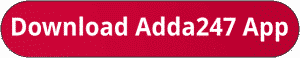Latest Banking jobs   »   Quantitative Aptitude Quiz For IBPS Clerk...

# Quantitative Aptitude Quiz For IBPS Clerk Prelims 2023 – 02nd July

Directions (1-5): Line graph given below shows the selling prices (in rupees) of three types of Refrigerators (A, B & C) in four different years i.e. 2016, 2017, 2018, and 2019 for a shopkeeperQ1. If a discount of 24% is given on refrigerator C sold in 2018 and ratio of MP to CP of C in 2018 is 5 : 3. then find the difference between the discount allowed and profit earned on C in 2018. (in rupees)
(a) 5000
(b) 4000
(c) 2000
(d) 6000
(e) 3000

Q2. Find out average selling price of refrigerator A in all the given years. (in rupees)
(a) 16500
(b) 22500
(c) 18500
(d) 19500
(e) 25500

Q3. Find out the ratio between selling price of refrigerator C in 2018 and the selling price of refrigerator A in 2017?
(a) 20:21
(b) 18:25
(c) 19:25
(d) 23:27
(e) 16:25

Q4. In which year sum of selling price of all 3 types of the refrigerator was the lowest?
(a) 2019
(b) 2016
(c) 2017
(d) 2018
(e) 2016 and 2018

Q5. selling price of refrigerator A in year 2018 is approx. what percent of selling price of refrigerator B in 2019?
(a) 78%
(b) 88%
(c) 82%
(d) 72%
(e) 93%

Direction (6-10): In each of these questions, two equations (I) and (II) are given. You have to solve both the equations and give the answers accordingly.

Q6.  I. x² – 14x + 48 = 0
II. y² – 17y + 72 =0

(a) if x > y
(b) if x ≥ y
(c) if x < y
(d) if x ≤ y
(e) if x = y or no relation can be established between x and y.

Q7.   I. x² + 13x + 42 = 0
II . y² + 15y + 56 = 0

(a) if x > y
(b) if x ≥ y
(c) if x < y
(d) if x ≤ y
(e) if x = y or no relation can be established between x and y.

Q8.  I . x² + 8x + 12 = 0
II .  6y² + 13y + 6 = 0

(a) if x > y
(b) if x ≥ y
(c) if x < y
(d) if x ≤ y
(e) if x = y or no relation can be established between x and y.

Q9.  2x² + 9x + 9 = 0
y² + 28y + 192 = 0

(a) if x > y
(b) if x ≥ y
(c) if x < y
(d) if x ≤ y
(e) if x = y or no relation can be established between x and y.

Q10. I . x² – 9x + 20 = 0
II.  y² – 6y + 9 = 0

(a) if x > y
(b) if x ≥ y
(c) if x < y
(d) if x ≤ y
(e) if x = y or no relation can be established between x and y.

Solutions## FAQs

### What is the selection process of IBPS Clerk 2023

The selection process of IBPS Clerk includes Prelims and Mains exam

#### Congratulations!Union Budget 2023-24: Free PDF